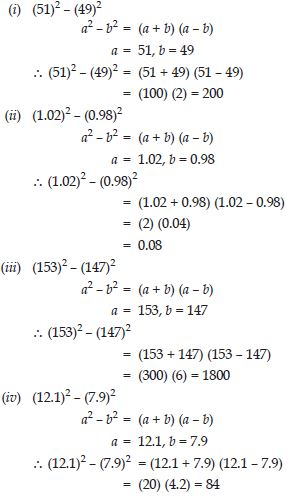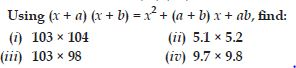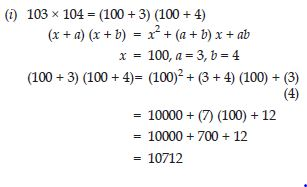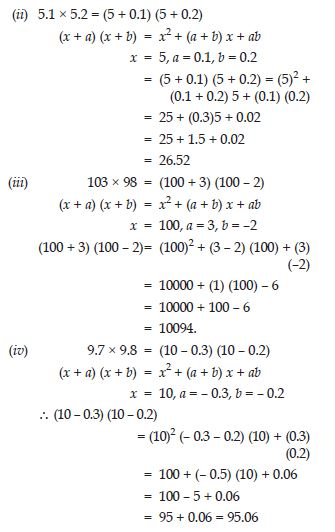# NCERT Solutions for Class 8 Math Chapter 9 - Algebraic Expressions and Identities

##### Question 1:

Give five examples of expressions containing one variable and five examples of expressions containing two variables.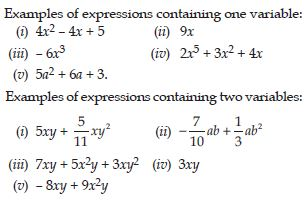##### Question 2:

Show on the number line x, x - 4, 2x + 1, 3x - 2.

Let us say the variable x has a position X on the number line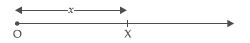The value of x - 4 will be 4 units to the left of X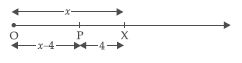The position of 2x will be point A, the distance of A from the origin will be twice the distance of X from the origin. The position B of 2x + 1 will be 1 unit to the right of A.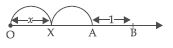The position of 3x will be point B; the distance of B from origin will be three times the distance of X from the origin. The position P of 3x - 2 will be 2 units to the left of B.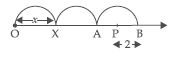##### Question 3:

Identify the coefficients of each term in the expression: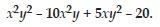Coefficient of x2y2 is 1
Coefficient of x2y is -10
Coefficient of xy2 is 5
Constant term is -20.

##### Question 4:

Classify the following polynomials as monomials, binomials, trinomials:
-z + 5, x + y + z, y + z + 100, ab - ac, 17.

Monomials: 17
Binomials: -z + 5, ab - ac
Trinomials: x + y + z, y + z + 100.

##### Question 5:

Construct 3 binomials with only x as a variable.

Binomials having x as a variable:
(i) 3x2 + 5x (ii) 6x3 + 2x (iii) 9x + 4x3

##### Question 6:

Construct 3 binomials with x and y as variable.

Binomials having x and y as variables:
(i) 3x2 y + 4xy (ii) 9xy + 6xy2 (iii) 5xy + 8x2 y

##### Question 7:

Construct 3 monomials with x and y as variables.

Monomials with x and y as variables:
(i) 4x2y (ii) 5xy2 (iii) 3xy

##### Question 8:

Construct 2 polynomials with 4 or more terms.

Polynomials having 4 or more terms:
(i) a + b + c + d
(ii) 2x3+ 5x2 + 3x + 18
(iii) 3x2 + 4xy + y2 + y + x + 9.

##### Question 9:

Write two terms which are like:
(i) 7xy (ii) 4 mn2 (iii) 2l.

(i) Terms which are like 7xy:
3xy, 9xy etc.
(ii) Terms which are like 4 mn2:
9mn2, mn2 etc.
(iii) Terms which are like 2l:
6l, 9l, 5l etc.

##### Question 10:

Identify the terms, their coefficients for each of the following expressions: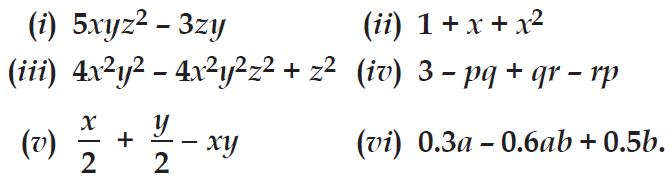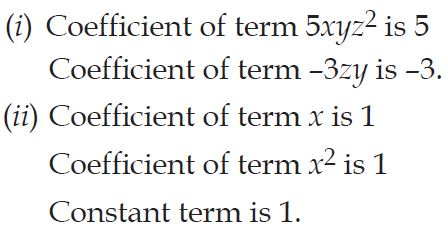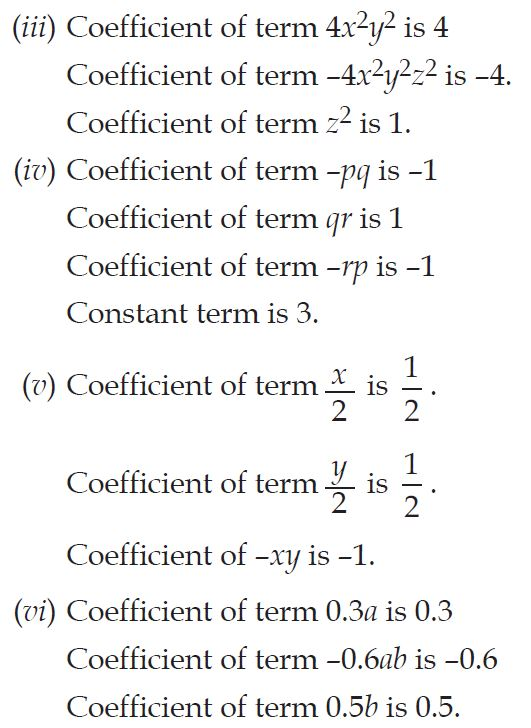##### Question 11:

Classify the following polynomials as monomials, binomials, trinomials. Which polynomials do not fit in any of these three categories?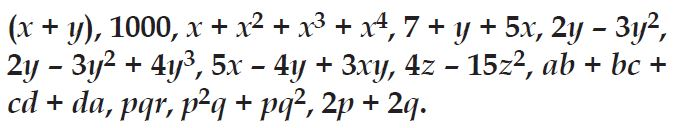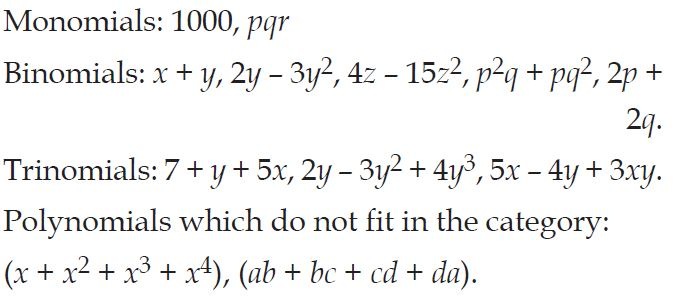##### Question 12:

ab - bc, bc - ca, ca - ab##### Question 13:

a - b + ab, b - c + bc, c - a + ac.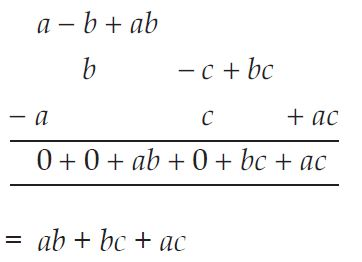##### Question 14:

2p2q2 - 3pq + 4, 5 + 7pq - 3p2q2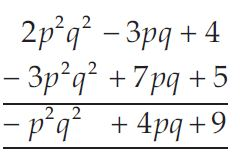##### Question 15:

l2 + m2, m2 + n2, n2 + l2, 2lm + 2mn + 2nl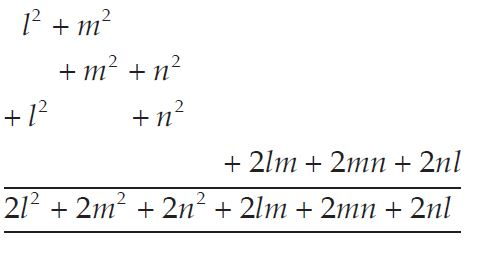##### Question 16:

Subtract 4a - 7ab + 3b +12 from 12a - 9ab + 5b - 3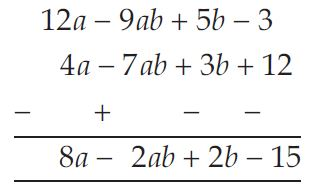##### Question 17:

Subtract 3xy + 5yz - 7zx from 5xy - 2yz - 2zx + 10xyz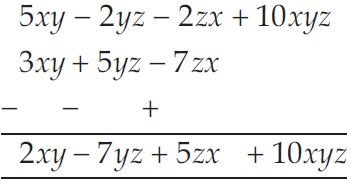##### Question 18:

Subtract 4p2q - 3pq + 5pq2 - 8p + 7q - 10 from
18 - 3p - 11q + 5pq - 2pq2 + 5p2q.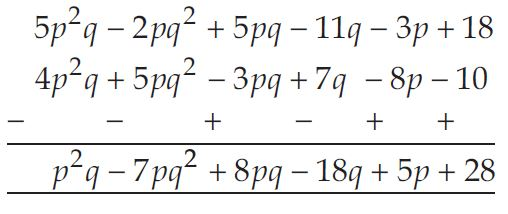##### Question 19:

Find 4x x 5y x 7z.
Does the order in which you carry out the multiplication matter?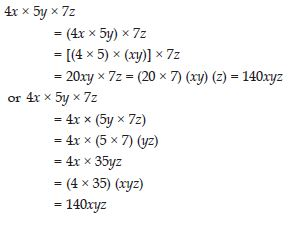The result is same in both the cases.
No, the order of multiplication does not alter the result.

##### Question 20:

Find the product of the following pairs of monomials:
(i) 4, 7p
(ii) -4p, 7p
(iii) -4p, 7pq
(iv) 4p3, -3p
(v) 4p, 0

(i) 4 x 7p = 28p
(ii) -4p x 7p = (-4 x 7) x (p x p) = -28p2
(iii) -4p x 7pq = (-4 x 7) x (p x pq) = -28p2q
(iv) 4p3 x -3p = [4 x (-3)] x (p3 x p) = -12p4
(v) 4p x 0 = 0.

##### Question 21:

Find the areas of rectangles with the following pairs of monomials as their lengths and breadths respectively:
(p, q); (10 m, 5n); (20x2, 5y2); (4x, 3x2); (3mn, 4np).

(i) Length of rectangle = p
Area of rectangle = length x breadth = p x q = pq.
(ii) Length of rectangle = 10 m
Area of rectangle = length x breadth = 10m x 5n
= 50mn.
(iii) Length of rectangle = 20x2
Area of rectangle = length x breadth
= 20x2 x 5y2
= 100x2y2.
(iv) Length of rectangle = 4x
Area of rectangle = length x breadth
= 4x x 3x2
= 12x3.
(v) Length of rectangle = 3mn
Area of rectangle = length x breadth = 3mn x 4np
= 12mn2p.

##### Question 22:

Complete the table of products: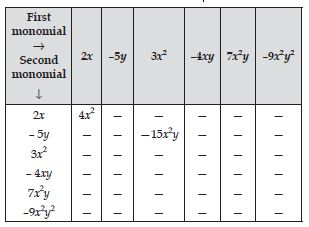Complete table of products is as under: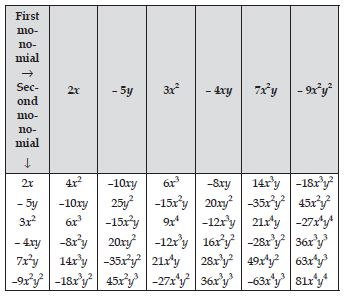##### Question 23:

Obtain the volume of rectangular boxes with the following length, breadth and height respectively.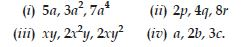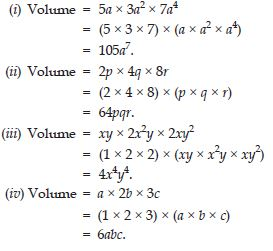##### Question 24: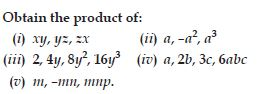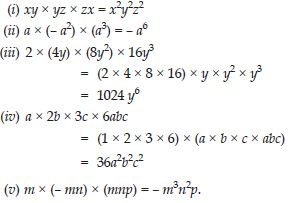##### Question 25:

Find the product:
(i) 2x(3x + 5xy) (ii) a2(2ab – 5c)

(i) (2x) x (3x + 5xy)
= (2x) x (3x) + (2x) x (5xy)
= 6x2 + 10x2y.
(ii) a2 (2ab – 5c)
= a2 x 2ab – a2 x 5c
= 2a3b – 5a2c.

##### Question 26:

Find the product: (4p2 + 5p + 7) x 3p.

4p2 x 3p + 5p x 3p + 7 x 3p
= 12p3 + 15p2 + 21p.

##### Question 27:

Carry out the multiplication of the expressions in each of the following pairs:
(i) 4p, q + r (ii) ab, a – b
(iii) a + b, 7a2b2 (iv) a2 – 9, 4a
(v) pq + qr + rp, 0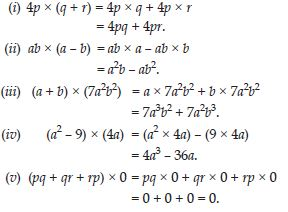##### Question 28:

Complete the table: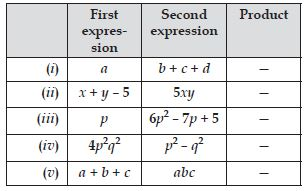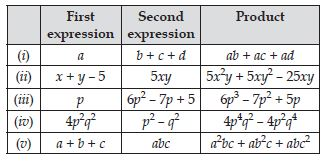##### Question 29: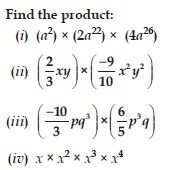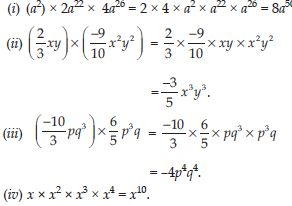##### Question 30: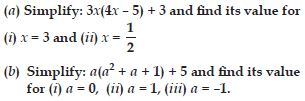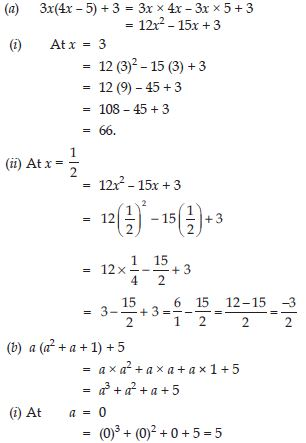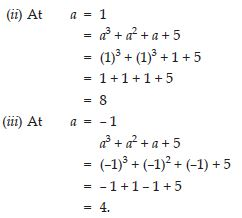##### Question 31: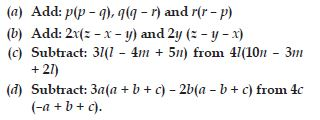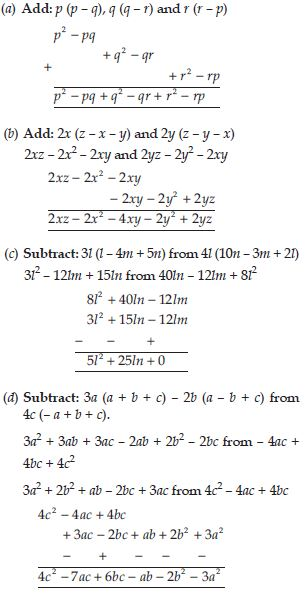##### Question 32:

Multiply the binomials: (2x + 5) and (4x – 3)

(2x + 5) x (4x – 3)
= 2x x (4x – 3) + 5 (4x – 3)
= 2x x 4x – 2x x 3 + 5 x 4x – 5 x 3
= 8x2 – 6x + 20x – 15
= 8x2 + 14x – 15

##### Question 33:

Multiply the binomials: (y – 8) and (3y – 4)

(y – 8) x (3y – 4)
= y (3y – 4) – 8 x (3y – 4)
= 3y2 – 4y – 24y + 32
= 3y2 – 28y + 32

##### Question 34:

Multiply the binomials: (2.5l – 0.5m) and (2.5l + 0.5m)

(2.5l – 0.5m) x (2.5l + 0.5m)
= 2.5l x (2.5l + 0.5m) – 0.5m (2.5l + 0.5m)
= 6.25l2 + 1.25lm – 1.25lm – 0.25m2
= 6.25l2 – 0.25m2

##### Question 35:

Multiply the binomials: (a + 3b) and (x + 5)

(a + 3b) x (x + 5)
= a x (x + 5) + 3b x (x + 5)
= ax + 5a + 3bx + 15b
= 5a + (a + 3b) x + 15b

##### Question 36:

Multiply the binomials: (2pq + 3q2) and (3pq – 2q2)

(2pq + 3q2) x (3pq – 2q2)
= 2pq x (3pq – 2q2) + 3q2 x (3pq – 2q2)
= 6p2q2 – 4pq3 + 9pq3 – 6q4
= 6p2q2 + 5pq3 – 6q4.

##### Question 37:

Multiply the binomials: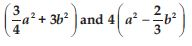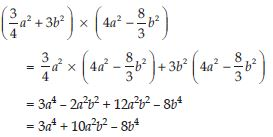##### Question 38:

Find the product: (5 – 2x) (3 + x)

(5 – 2x) (3 + x)
= 5 x (3 + x) – 2x x (3 + x)
= 15 + 5x – 6x – 2x2
= 15 – x – 2x2

##### Question 39:

Find the product: (x + 7y) (7x – y)

(x + 7y) (7x – y)
= x x (7x – y) + 7y x (7x – y)
= 7x2 – xy + 49xy – 7y2
= 7x2 + 48xy – 7y2

##### Question 40:

Find the product: (a2 + b) (a + b2)

(a2 + b) (a + b2)
= a2 (a + b2) + b (a + b2)
= a3 + a2b2 + ab + b3

##### Question 41:

Find the product: (p2 – q2) (2p + q)

(p2 – q2) (2p + q) = p2 (2p + q) – q2 (2p + q)
= 2p3 + p2q – 2pq2 – q3

##### Question 42:

Simplify:
(x2 – 5) (x + 5) + 25

(x2 – 5) (x + 5) + 25
= x2 (x + 5) – 5 (x + 5) + 25
= x3 + 5x2 – 5x – 25 + 25
= x3 + 5x2 – 5x

##### Question 43:

Simplify:
(a2 + 5) (b3 + 3) + 5

(a2 + 5) (b3 + 3) + 5
= a2 (b3 + 3) + 5 (b3 + 3) + 5
= a2b3 + 3a2 + 5b3 + 15 + 5
= a2b3 + 3a2 + 5b3 + 20

##### Question 44:

Simplify:
(t + s2) (t2 – s)

(t + s2) (t2 – s)
= t (t2 – s) + s2 (t2 – s)
= t3 – ts + s2t2 – s3

##### Question 45:

Simplify:
(a + b) (c – d) + (a – b) (c + d) + 2 (ac + bd)

(a + b) (c – d) + (a – b) (c + d) + 2 (ac + bd)
= a(c – d) + b(c – d) + a(c + d) – b(c + d) + 2ac + 2bd
= ac – ad + bc – bd + ac + ad – bc – bd + 2ac + 2bd
= 4ac

##### Question 46:

Simplify:
(x + y) (2x + y) + (x + 2y) (x – y)

(x + y) (2x + y) + (x + 2y) (x – y)
= x(2x + y) + y(2x + y) + x(x – y) + 2y(x – y)
= 2x2 + xy + 2xy + y2 + x2 – xy + 2xy – 2y2
= 3x2 + 4xy – y2

##### Question 47:

Simplify:
(x + y) (x2 – xy + y2)

(x + y) (x2 – xy + y2)
= x (x2 – xy + y2) + y (x2 – xy + y2)
= x3 – x2y + xy2 + yx2 – xy2 + y3
= x3 + y3

##### Question 48:

Simplify:
(1.5x – 4y) (1.5x + 4y + 3) – 4.5x + 12y

(1.5x – 4y) (1.5x + 4y + 3) – 4.5x + 12y
= 1.5x(1.5x + 4y + 3) – 4y (1.5x + 4y + 3) – 4.5x + 12y
= 2.25x2 + 6xy + 4.5x – 6xy – 16y2 – 12y – 4.5x + 12y
= 2.25x2 – 16y2

##### Question 49:

Simplify:
(a + b + c) (a + b – c)

(a + b + c) (a + b – c)
= a (a + b – c) + b (a + b – c) + c (a + b – c)
= a2 + ab – ac + ab + b2 – bc + ac + bc – c2
= a2 + b2 – c2 + 2ab

##### Question 50:

Put –b in place of b in Identity (i): (a + b)2 = a2 + 2ab + b2. Do you get Identity (ii): (a – b)2 = a2 – 2ab + b2?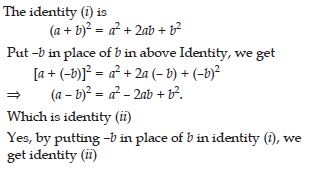##### Question 51:

Verify identity (iv): (x + a)(x + b) = x2 + x(a + b) + ab for a = 2, b = 3, x = 5.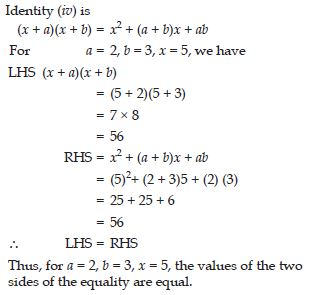##### Question 52:

Consider, the special case of identity (iv): (x + a)(x + b)= x2 + x(a + b) + ab with a = b, what do you get? Is it related to Identity (i): (x + a)2 = x2 + 2ax + a2?

The Identity (iv) is
(x + a) (x + b) = x2 + (a + b)x + ab
For a = b the above identity becomes
(x + a)(x + a) = x2 + (a + a) x + a.a
$⇒$ (x + a)2 = x2 + 2ax + a2
Yes, it is related to identity (x + a)2 = x2 + 2ax + a2.

##### Question 53:

Consider, the special case of identity (iv): (x + a)(x + b)= x2 + x(a + b) + ab with a = –c and b = –c. What do you get? Is it related to Identity (ii): (x – c)2 = x2 – 2cx + c2?

The Identity (iv) is
(x + a) (x + b) = x2 + (a + b) x + ab
For a = – c and b = – c the identity (iv) becomes
[x + (–c)][x + (– c)] = x2 + [( – c) + (–c)]x + (– c)(– c)
$⇒$ (x – c) (x – c) = x2 + ( –2c) x + c2
$⇒$ (x – c) (x – c) = x2 – 2 cx + c2
$⇒$ (x – c)2 = x2 – 2cx + c2
Yes, it is related to identity (ii).

##### Question 54:

Consider the special case of identity (iv): (x + a)(x + b)= x2 + x(a + b) + ab with b = –a. What do you get? Is it related to identity (iii): (x – c)2 = x2 – 2cx + c2.

The identity (iv) is
(x + a) (x + b) = x2 + (a + b) x + ab
For b = – a, identity (iv) becomes
(x + a) [x + (– a)] = x2 + [(a) + (–b)]x + (a)(– a)
$⇒$ (x + a) (x – a) = x2 + (a – a)x – a2
$⇒$ (x + a) (x – a) = x2 + (0)x – a2
$⇒$ (x + a) (x – a) = x2 – a2.
Yes, it is related to identity (iii): (x – c)2 = x2 – 2cx + c2.

##### Question 55:

Use a suitable identity to get product of (x + 3) (x + 3)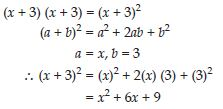##### Question 56:

Use a suitable identity to get product of (2y + 5) (2y + 5)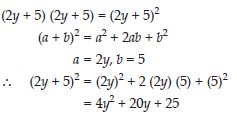##### Question 57:

Use a suitable identity to get product of (2a – 7) (2a – 7)##### Question 58:

Use a suitable identity to get product of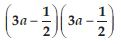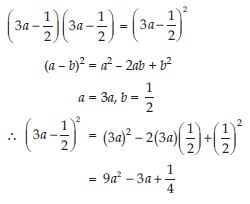##### Question 59:

Use a suitable identity to get product of (1.1m – 0.4) (1.1m + 0.4)

(1.1m – 0.4) (1.1m + 0.4)
(a + b) (a – b) = a2 – b2
a = 1.1m, b = 0.4
$\therefore$ (1.1m – 0.4) (1.1m + 0.4) = (1.1m)2 – (0.4)2
= 1.21m2 – 0.16

##### Question 60:

Use a suitable identity to get product of
(a2 + b)2 (– a2 + b2)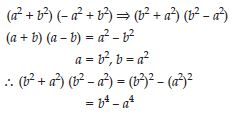##### Question 61:

Use a suitable identity to get product of (6x – 7) (6x + 7)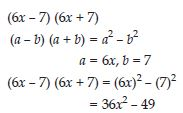##### Question 62:

Use a suitable identity to get product of (– a + c) (– a + c)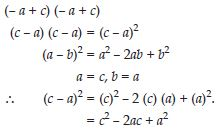##### Question 63:

Use a suitable identity to get each of the following products: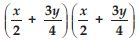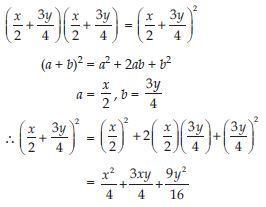##### Question 64:

Use a suitable identity to get product of (7a – 9b) (7a – 9b)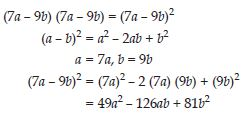##### Question 65:

Use the identity (x + a) (x + b) = x2 + (a + b) x + ab to find the following product: (x + 3) (x + 7)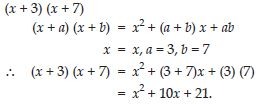##### Question 66:

Use the identity (x + a) (x + b) = x2 + (a + b) x + ab to find the following product: (4x + 5) (4x + 1)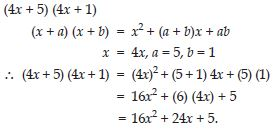##### Question 67:

Use the identity (x + a) (x + b) = x2 + (a + b) x + ab to find the following product: (4x – 5) (4x – 1)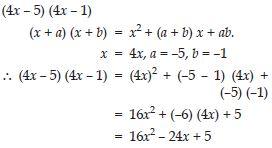##### Question 68:

Use the identity (x + a) (x + b) = x2 + (a + b) x + ab to find the following product: (4x + 5) (4x – 1)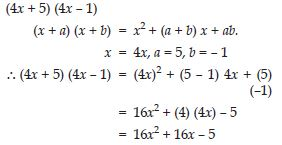##### Question 69:

Use the identity (x + a) (x + b) = x2 + (a + b) x + ab to find the following product: (2x + 5y) (2x + 3y)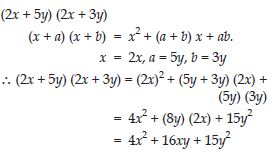##### Question 70:

Use the identity (x + a) (x + b) = x2 + (a + b) x + ab to find the following product: (2a2 + 9) (2a2 + 5)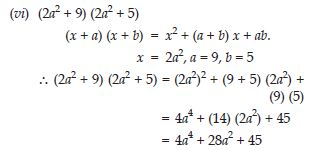##### Question 71:

Use the identity (x + a) (x + b) = x2 + (a + b) x + ab to find the following product: (xyz – 4) (xyz – 2)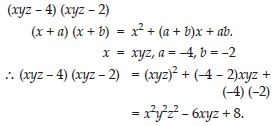##### Question 72: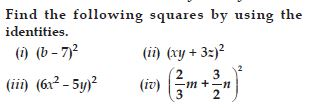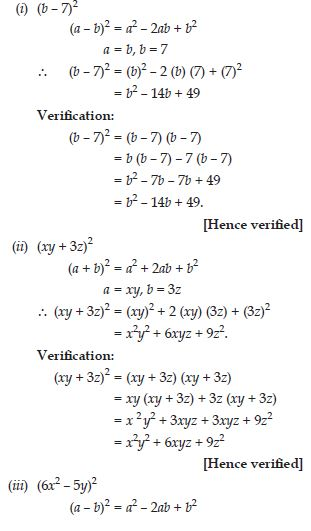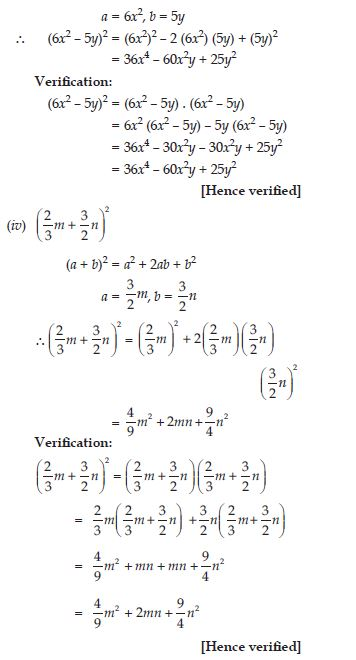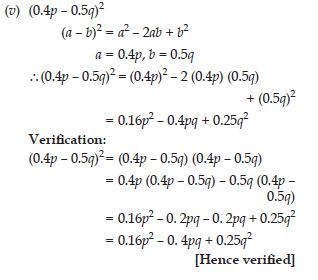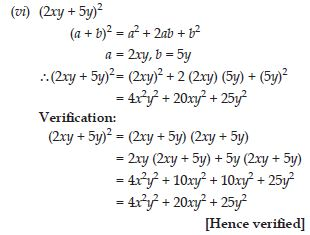##### Question 73: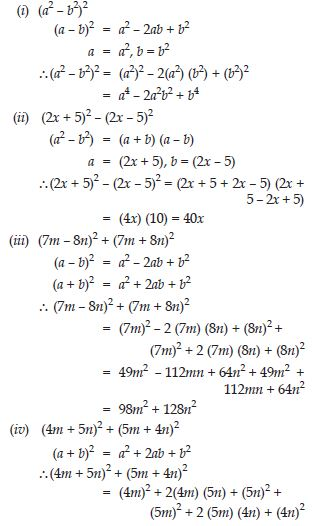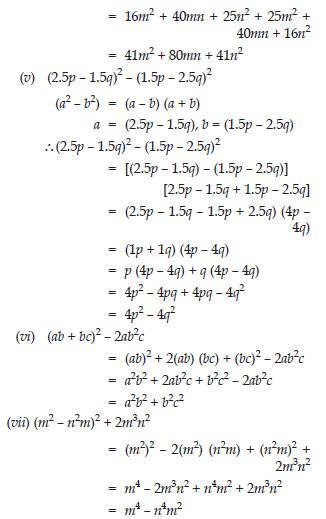##### Question 74: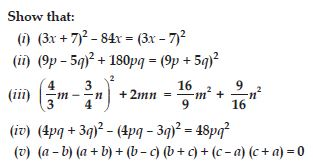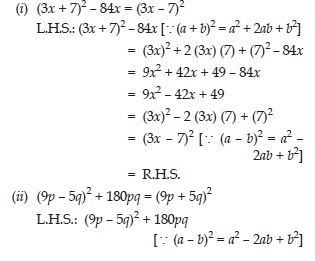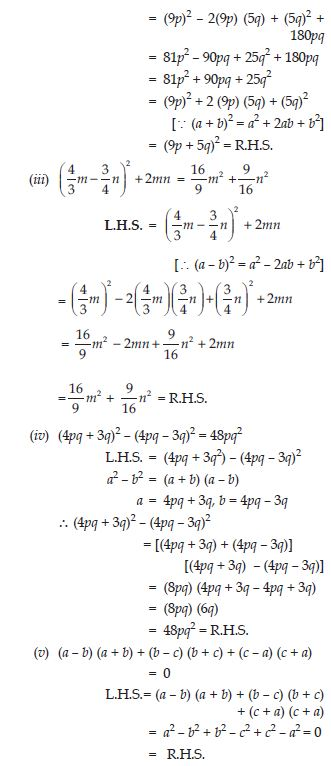##### Question 75: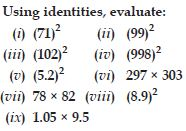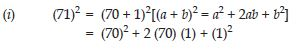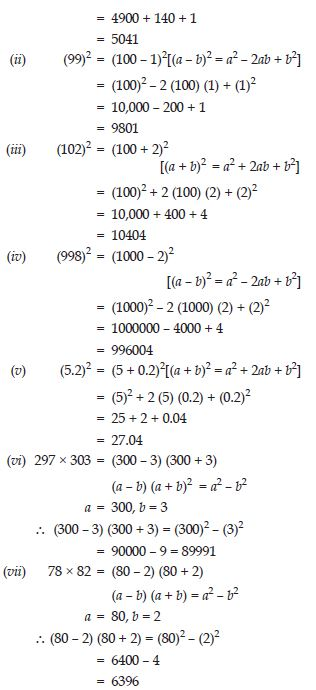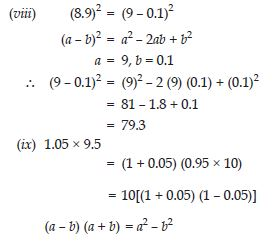a = 1, b = 0.05
$⇒$ (1 + 0.05) (1 – 0.05)
= (1)2 – (0.05)2
= 1 – 0.0025 = 0.9975
$\therefore$ 1.05 × 9.5 = 10 × 0.9975 = 9.975

##### Question 76: